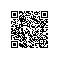# SQL调优指南—SQL调优进阶—排序优化和执行

## 排序（Sort）

PolarDB-X中的排序算子主要包括 MemSort、TopN，以及 MergeSort。

MemSort

PolarDB-X中的通用的排序实现为MemSort算子，即内存中运行快速排序（Quick Sort）算法。下面是一个用到MemSort算子的例子：

> explain select t1.name from t1 join t2 on t1.id = t2.id order by t1.name,t2.name;
Project(name="name")
MemSort(sort="name ASC,name0 ASC")
Project(name="name", name0="name0")
BKAJoin(condition="id = id", type="inner")
Gather(concurrent=true)
LogicalView(tables="t1", shardCount=2, sql="SELECT id, name FROM t1 AS t1")
Gather(concurrent=true)
LogicalView(tables="t2_[0-3]", shardCount=4, sql="SELECT id, name FROM t2 AS t2 WHERE (id IN ('?'))")

TopN

TopN算子维护一个最大或最小堆，按照排序键的值，堆中始终保留最大或最小的N行数据。当处理完全部的输入数据时，堆中留下的N个行（或小于N个）就是需要的结果。

> explain select t1.name from t1 join t2 on t1.id = t2.id order by t1.name,t2.name limit 10;
Project(name="name")
TopN(sort="name ASC,name0 ASC", offset=0, fetch=?0)
Project(name="name", name0="name0")
BKAJoin(condition="id = id", type="inner")
Gather(concurrent=true)
LogicalView(tables="t1", shardCount=2, sql="SELECT id, name FROM t1 AS t1")
Gather(concurrent=true)
LogicalView(tables="t2_[0-3]", shardCount=4, sql="SELECT id, name FROM t2 AS t2 WHERE (id IN ('?'))")

MergeSort

> explain select name from t1 order by name;
MergeSort(sort="name ASC")
LogicalView(tables="t1", shardCount=2, sql="SELECT name FROM t1 AS t1 ORDER BY name")